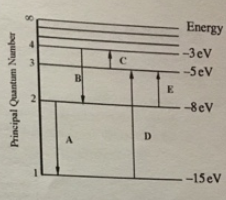# Problem: For this question consider the Figure to the right shows an energy level diagram for a certain atom (not hydrogen). Several transitions are shown and are labelled by letters. Note: The diagram is not drawn to scale. Which transition corresponds to the emission of a 177 nm photon?

###### FREE Expert Solution

The energy of a photon:

$\overline{){\mathbf{E}}{\mathbf{=}}{\mathbf{h}}{\mathbf{f}}{\mathbf{=}}\frac{\mathbf{h}\mathbf{c}}{\mathbf{\lambda }}}$

Ephoton = hc/λ = (6.62 × 10-34)(3.0 × 108)/(177 × 10-9

98% (287 ratings)###### Problem Details

For this question consider the Figure to the right shows an energy level diagram for a certain atom (not hydrogen). Several transitions are shown and are labelled by letters. Note: The diagram is not drawn to scale.Which transition corresponds to the emission of a 177 nm photon?